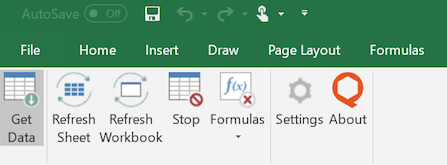The Excel Add-In works with Windows and the desktop versions of Excel 2013, 2016 and 2019. The Excel Add-In is not compatible with online versions of Excel, and it is not currently compatible with Mac.

When using the Excel Add-In, note that data on Quandl comes in two formats:  time-series and tables. You can learn more about these formats here. Below are instructions on how to download time-series and tables data using the Excel Add-In.

Although very rare, a few data feeds use a custom API and do not use the API for time-series or tables. These data feeds may not be downloaded through the Excel Add-In, and Quandl's Python Package and R Package.

Each data feed that uses a custom API comes with documentation that explains how to make API calls for that feed. For example, the  ORATS Smoothed Options Market Quotes data feed uses a custom API and its  Documentation explains how to make custom API calls. Data feeds using a custom API may only be downloaded via the custom API routes described in their documentation.

Time-series
Once the Excel Add-In is installed, you can download time-series data by using the Quandl Formula Builder or by using the QSERIES formula

To use the Quandl Formula Builder, simply open Excel, go to the "Quandl" tab and click the "Get Data" button.Here is a short tutorial that explains how to use the Quandl Formula Builder with time-series data:

To learn how to use the QSERIES formula, see the documentation here.

Here is a short tutorial that explains how to use the QSERIES formula:

Tables
Once the Excel Add-In is installed, you can retrieve data from tables by using the Quandl Formula Builder or by using the QTABLE formula.

To use the Quandl Formula Builder, simply open Excel, go to the "Quandl" tab and click the "Get Data" button.Here is a short tutorial that explains how to use the Quandl Formula Builder with data from tables:

To learn how to use the QTABLE formula, see the documentation here.

Here is a short tutorial that explains how to use the QTABLE formula: